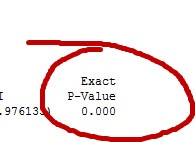Statistics can be confusing, especially when you look under the hood at the mathematical engines that underlie it. That's why we use statistical software to do so much of the work for us, and why we use tools like p-values to help us make sense of what our data are saying.

The p-value is used in basic statistics, linear models, reliability, multivariate analysis, and many other methods. It's a concept every introductory statistics student and every Lean Six Sigma Green Belt learns at the start.  But it's frequently misinterpreted.

Andrew Gelman, director of the Applied Statistics Center at Columbia University, wrote a blog post that contains (amidst other interesting discussion) a good explanation of what a p-value is and, probably even more important, is NOT:

"A p-value is the probability of seeing something as extreme as was observed, if the model were true."

In hypothesis testing, when your p-value is less than the alpha level you selected (typically 0.05), you'd reject the null hypothesis in favor of the alternative hypothesis.

Let's say we do a 2-sample t-test to assess the difference between the mean strength of steel from two mills. The null hypothesis says the two means are equal; the alternative hypothesis states that they are not equal.

If we get a p-value of 0.02 and we're using 0.05 as our alpha level, we would reject the hypothesis that the population means are equal.

But here are three things we can't say based on the p-value:

1. "There is 2% probability no difference exists, and 98% probability it does."
In fact, the p-value only says that IF the null hypothesis were true, we would see a difference as large or larger than this one only 2% of the time. If this seems confusing, just keep in mind that the p-value doesn't tell you anything directly about what you're observing, it tells you about your odds of observing it.
2. "Since we have a low p-value, this difference is important."
A p-value can tell you that a difference is statistically significant, but it tells you nothing about the size or magnitude of the difference.
3. "The p-value is low, so the alternative hypothesis is true."
A low p-value can give us a statistical evidence to support rejecting the null hypothesis, but it does not prove that the alternative hypothesis is true. If you use an alpha level of 0.05, there's a 5% chance you will incorrectly reject the null hypothesis.

Does this mean that quality practitioners and others shouldn't use p-values?  Of course not--the p-value is a very useful tool!  We just need to be careful about how we interpret the p-value, and particularly careful about how we explain its significance to others.